Back to Adaptive Vision Studio website

You are here: Start » Filter Reference » Geometry 2D Features

# Geometry 2D Features

Select a filter from the list below.

Icon Name Description Modules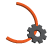ArcCircle Computes the circle containing an arc. FoundationLiteArcEndpoints Computes two endpoints of an arc. FoundationLiteArcLength Computes the length of an arc. FoundationLiteArcMidpoint Computes the midpoint of an arc. FoundationLite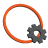CircleArea Computes the area of a circle. FoundationLiteCircleBoundingBox Computes the smallest box containing a circle. FoundationLiteCircleBoundingRectangle Computes the smallest rectangle containing a circle. FoundationLiteCircleCharacteristicPoint Returns a characteristic point (e.g. the top-left) of a box containing the input circle. FoundationLiteCirclePerimeterLength Computes the length of a circle perimeter. FoundationLiteCircleSection Computes an arciform section of the circle perimeter. FoundationLite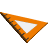LineNormalVector Computes the normal vector of a line FoundationLiteLineOrientation Computes the orientation angle of a line as angle in the range from 0 to 180. FoundationLite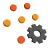PointsBoundingBox Computes the smallest box containing an array of points. FoundationLitePointsBoundingBox_OrNil Computes the smallest box containing an array of points; returns NIL if the array is empty. FoundationLitePointsBoundingCircle Computes the smallest circle containing an array of points. FoundationBasicPointsBoundingCircle_OrNil Computes the smallest circle containing an array of points; returns NIL if the array is empty. FoundationBasicPointsBoundingParallelogram Computes the smallest parallelogram containing an array of points. FoundationProPointsBoundingRectangle Computes the smallest rectangle containing an array of points. FoundationLitePointsBoundingRectangle_FixedAngle Computes the smallest rectangle with the given orientation angle containing an array of points. FoundationLitePointsBoundingRectangle_FixedAngle_OrNil Computes the smallest rectangle with the given orientation angle containing an array of points; returns NIL when the array is empty. FoundationLitePointsBoundingRectangle_OrNil Computes the smallest rectangle containing an array of points; returns NIL when the array is empty. FoundationLitePointsCaliperDiameter Computes the longest and the shortest width of the input points measured as distance between parallel lines containing all of them. FoundationBasicPointsConvexHull Computes the smallest convex shape that contains the given array of points. FoundationLitePointsDiameter Finds the longest segment connecting two points from a given array. FoundationLitePointsMassCenter Computes the central point of the input points. FoundationLitePointsMassCenter_OrNil Computes the central point of the input points; returns NIL if the array is empty. FoundationLitePointsMedian Computes the geometric median of the input points. FoundationLitePointsOrientation Computes the orientation of a set of 2D points. FoundationLite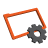RectangleArea Computes the area of a rectangle. FoundationLiteRectangleBoundingBox Computes the smallest box containing a rectangle. FoundationLiteRectangleBoundingCircle Computes the smallest circle containing a rectangle. FoundationLiteRectangleCenter Computes the center point of a rectangle. FoundationLiteRectangleCharacteristicPoint Returns a characteristic point (e.g. the top-left) of the input rectangle. FoundationLiteRectangleCharacteristicPoints Computes rectangle's characteristic points. FoundationLiteRectangleCorners Computes the four corner points of a rectangle. FoundationLiteRectanglePerimeterLength Computes the perimeter length of a rectangle. FoundationLite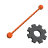SegmentBisector Computes a line passing through the center of a segment at a right angle. FoundationLiteSegmentCenter Computes the center point of a segment. FoundationLiteSegmentLength Computes the length of a segment. FoundationLiteSegmentLine Computes the line passing through a segment. FoundationLiteSegmentNormalVector Computes an unitary vector perpendicular to the segment. FoundationLiteSegmentOrientation Computes the orientation of a segment. FoundationLiteSegmentVector Returns the vector [x2 - x1, y2 - y1] FoundationLite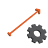VectorDirection Computes the direction angle of a vector as an angle in range the from 0 to 360. FoundationLiteVectorLength Computes the length of a vector. FoundationLiteVectorsMedian Computes the geometric median of the input vectors. FoundationLite